# RD Sharma Class 8 Solutions Chapter 27 Introduction to Graphs Ex 27.1

## RD Sharma Class 8 Solutions Chapter 27 Introduction to Graphs Ex 27.1

These Solutions are part of RD Sharma Class 8 Solutions. Here we have given RD Sharma Class 8 Solutions Chapter 27 Introduction to Graphs Ex 27.1

Other Exercises

Question 1.
Plot the points (5,0 ), (5,1), (5, 8). Do they lie on a line ? What is your observation ?
Solution:
Draw XOX’ and YOY’ the co-ordinates axis on the graph.
Take 1 cm = 1 unit
Point A (5, 0), B (5, 1) and C (5, 8) have been plotted on the graph. By joining A, B and C, we see that these points lie on the same line which is 5 units from y-axis.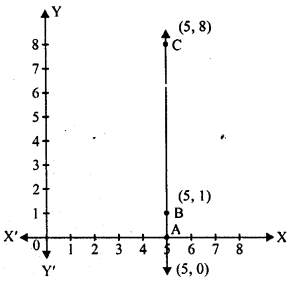Question 2.
Plot the points (2, 8), (7, 8) and (12, 8). Join these points in pairs. Do they lie on a line ? What do you observe ?
Solution:
Draw XOX’ and YOY’, the co-ordinate axis on the graph.
Take 0.5 cm = 1 unit.
Now points A (2, 8), B (7, 8), and C (12, 8) have been plotted on the graph. By joining them, we see that these points lie on the same line which is at a distance of 8 unit from x-axis.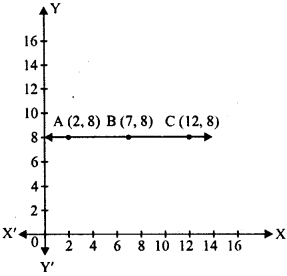Question 3.
Locate the points :
(i) (1,1), (1,2), (1,3), (1,4)
(ii) (2,1), (2, 2), (2,3), (2,4)
(iii) (1,3), (2,3), (3,3), (4,3)
(iv) (1,4), (2,4), (3,4), (4,4).
Solution:
The points given in (i) and (ii) are locates in first graph.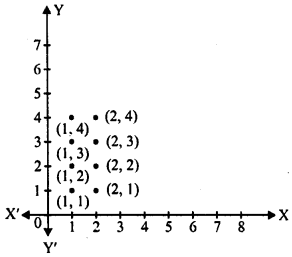(i) (1,1), (1,2), (1,3), (1,4)
(ii) (2,1), (2, 2), (2, 3), (2, 4)
Points of (iii) and (iv) are located in the adjoining graph.
(iii) (1, 3), (2, 3), (3, 3), (4, 3)
(iv) (1,4), (2, 4), (3, 4), (4,4)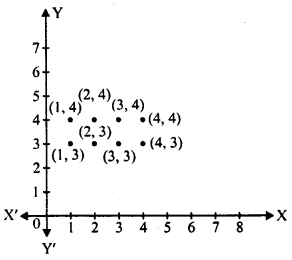Question 4.
Find the coordinates of points A, B, C, D in the figure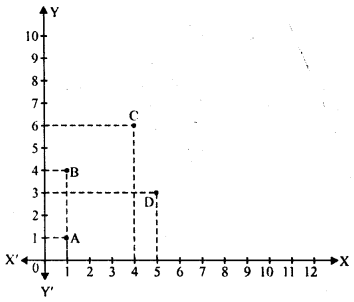Solution:
Draw perpendicular from A, B, C and D on x-axis and also on y-axis.
A is 1 unit from y-axis and 1 unit from x-axis.
∴ Co-ordinates of A are (1,1)
B is 1 unit fr onr y-axis and 4 units from x-axis
∴ Co-ordinates of B are (1,4)
C is 4 units from y-axis and 6 units from x-axis
∴ Co-ordinates of C are (4, 6)
D is 5 units fromy-axis and 3 units from x-axis
∴ Co-ordinates of D are (5,3)

Question 5.
Find the coordinates of points P, Q, R and S in Fig.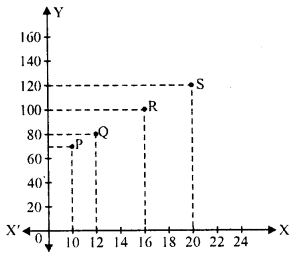Solution:
Through P, Q, R and S, draw perpendiculars on x-axis and also on y-axis.
(i) P is 10 units form >’-axis and 70 units from y-axis
∴ Co-ordinates of P are (10, 70)
(ii) Q is 12 unit from x-axis and 80 units from y-axis
∴ Co-ordinates of Q are (12, 80)
(iii) R is 16 units from x-axis and 100 units from y-axis
∴ Co-ordinates of R are (16, 100)
(iv) S is 20 units from x-axis and 120 units from y-axis
∴ Co-ordinates of S are (20, 120)

Question 6.
Write the coordinates of each of the vertices of each polygon in the figure.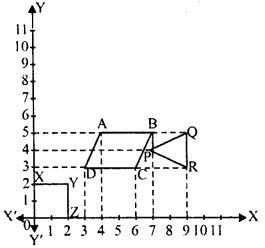Solution:
(i) In figure OXYZ
Co-ordinates of O are (0, 0) ∵It is the origin
Co-ordinates of X are (0, 2) ∵ It lies on y – axis
Co-ordinates of Y are (2, 2)
Co-ordinates of Z are (2, 0) ∵It lies on x-axis
(ii) In figure ABCD, draw perpendicular from A, B, C, D on x-axis and y-axis.
A is 4 unit from y-axis and 5 units from x- axis
∴ Co-ordinates of A are (4, 5)
B is 7 units from y-axis and 5 units from x – axis
∴ Co-ordinates of B are (7, 5)
C is 6 units from y-axis and 3 units from x- axis
∴ Co-ordinates of C are (6, 3)
D is 3 units from y-axis as well x-axis
∴ Co-ordinates of D are (3, 3)
(iii) In figure PQR, perpendiculars for P, Q, R are drawn on x-axis and also on y-axis.
∴ Co-ordinates of P are (7, 4), of Q are (9, 5) and of R are (9, 3).

Question 7.
Decide which of the following statements is true and which is false. Give reasons for your answer.
(i) A point whose x-coordinate is zero, will lie on they-axis.
(ii) A point whose y-coordinate is zero, will lie on x-axis.
(iii) The coordinates of the origin are (0, 0).
(iv) Points whose x and y coordinates are equal, lie on a line passing through the origin.
Solution:
(i) Correct: ∵ every point on y-axis, its x = 0
(ii) Correct: ∵ every point on x-axis, its y = 0
(iii) Correct
(iv) Correct

Hope given RD Sharma Class 8 Solutions Chapter 27 Introduction to Graphs Ex 27.1 are helpful to complete your math homework.

If you have any doubts, please comment below. Learn Insta try to provide online math tutoring for you.